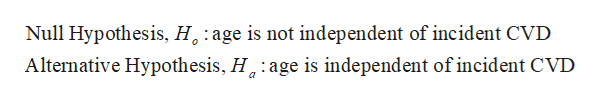# Age Incident CVDNo CVDTotal< 504555560050+61339400Total1068941000Is age independent of incident CVD? Preform a chi-squared test of independence. Remember to document the 5 steps used in hypothesis testing. Why is age a confounding factor in this example?

Question
6 views
 Age Incident CVD No CVD Total < 50 45 555 600 50+ 61 339 400 Total 106 894 1000

Is age independent of incident CVD? Preform a chi-squared test of independence. Remember to document the 5 steps used in hypothesis testing. Why is age a confounding factor in this example?

check_circle

Step 1

Given data table in the this question is,

 Age Incident CVD No CVD Total < 50 45 555 600 50+ 61 339 400 Total 106 894 1000

Step 2

The hypothesis is as:help_outlineImage TranscriptioncloseNull Hypothesis, H age is not independent of incident CVD Alternative Hypothesis, H:age is independent of incident CVD fullscreen
Step 3

Number of rows (r) = 2

Number of columns (c) = 2

Now, degre...

### Want to see the full answer?

See Solution

#### Want to see this answer and more?

Solutions are written by subject experts who are available 24/7. Questions are typically answered within 1 hour.*

See Solution
*Response times may vary by subject and question.
Tagged in

### Statistics